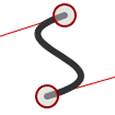# How to Draw Cubic Bézier Curves on HTML5 SVGs

In my previous SVG posts, we examined the path element and quadratic bézier curves with a single control point. In this post, we’re going to examine the second of three types: the cubic bézier curve.

## What are Cubic Bézier Curves?

You’ve probably encountered cubic béziers in desktop publishing and graphics packages. So let’s take another trip to WolframMathWorld to look at the equations. Ugghh.In the last post, we saw how quadratic béziers have a start point (P0), end point (P2) and a single control point (P1) which determines the curvature. Cubic bézier curves also have start (P0) and end points (P3), but there are two control points — one for each end of the line (P1 and P2). Wikipedia illustrates how they are generated.Bézier provides far more curve options. With two control points we can generate curves which reverse direction or wrap around themselves.

## Path Puzzles

Quadratic bézier curves are defined using the ‘C’ directive in the path’s ‘d’ attribute:

``````
<path d="M100,250 C100,100 400,100 400,250" />
``````

The initial M directive moves the pen to the first point (100,250). Following the ‘C’ are three co-ordinates; the start control point (100,100), the end control point (400,100) and the final point we’re drawing to (400,250).

You can also use a lowercase ‘c’ to denote relative rather than absolute co-ordinates. The following curve is identical:

``````
<path d="M100,250 c0,-150 300,-150 300,0" />
``````

Again, there are shorthand ‘S’ and ‘s’ directives. These accept two co-ordinates; the final point and its associated control point. The first control point is assumed to be the same as the end control point of the previous curve, e.g.

``````
<path d="M100,250 C100,100 400,100 400,250 S400,400 250,250" />
``````

This draws a curve from 100,250 (control point at 100,100) to 400,250 (control point at 400,100). Another curve is then drawn from 400,250 (control point unchanged at 400,100) to 250,250 (control point at 400,400).

As usual, the lowercase ‘s’ directive denotes relative rather than absolute co-ordinates.

Fortunately, you don’t need to worry too much about all the confusing co-ordinates; visit the interactive SVG cubic bézier curve demonstration page — it works in all browsers except IE8 and below which don’t support SVGs.

Drag the control points or either end of the curve and the code is generated for you. Click the curve to toggle a fill effect.

In the next SVG post, we’ll complete our path element series with elliptical arcs.